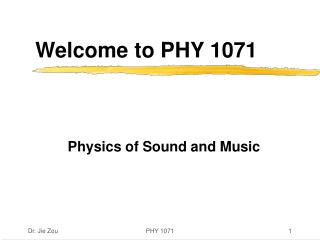Download PresentationWelcome to PHY 1071

# Welcome to PHY 1071

Download Presentation## Welcome to PHY 1071

- - - - - - - - - - - - - - - - - - - - - - - - - - - E N D - - - - - - - - - - - - - - - - - - - - - - - - - - -
##### Presentation Transcript

1. Welcome to PHY 1071 Physics of Sound and Music PHY 1071

2. 2Newton’s First Law of Motion • Newton’s First Law of Motion-Inertia • Net Force • Support Force • The Equilibrium Rule • Inertia and the Moving Earth PHY 1071

3. Newton’s 1st Law of Motion • The law of inertia: • Every object continues in its state of rest, or of uniform motion in a straight line, unless it is compelled to change that state by forces impressed upon it. • Continues: an object continues to do whatever it happens to be doing unless an (unbalanced) force exerted upon it. • Inertia: • The property of objects to resist changes in motion. PHY 1071

4. Understanding Inertia • Inertia: the property of things to resist changes in motion. • In the absence of external forces, a body at rest tends to remain at rest; if moving, it tends to remain moving. • Once a body is in motion, no force is needed to keep it moving in the absence of retarding force, such as friction. • Inertia is a property of matter to behave this way. It is not some kind of force that keeps things moving. • A force is needed to change motion (e.g. changing the direction or speed of the motion), not to keep a body moving, so long as friction is negligible. PHY 1071

5. STOP Keeps moving Suddenly slows down. Standing in a moving bus How about when the bus suddenly speeds up? Examples of Inertia • You see inertia everywhere! • Example 1: standing in a moving bus The bus suddenly slows down. Do you lurch forward or backward? And why? PHY 1071

6. Applied forces Net force 5 N 10 N 5 N 0 N 5 N 5 N 5 N 5 N 10 N Net Force • Force: • Changes in motion are produced by a force or combination of forces. • A force, in the simplest sense, is a push or a pull. • Net force: • When more than a single force acts on an object, we consider the net force; force is a vector quantity-has both magnitude and direction. PHY 1071

7. N=G or N + (-G) = 0 F=0 N Book G Support Force • It is a normal force: force that acts at right angles to the surface. • It is a very real force. • Examples of support force: • A book supported by the table. • A person supported by a weight scale. • Units of force: the Newton (N), pound • Some common forces: Gravity (G), Normal force (N) PHY 1071

8. The Equilibrium Rule • Mechanical equilibrium: • When the net force on something is zero, we say something is in mechanical equilibrium. • Equilibrium is a state of no change. • Forms of mechanical equilibrium: • Rest is only one form of equilibrium. • Uniform motion (constant speed in a straight line). • Rotational equilibrium (Ch.8). • The equilibrium rule: • For any object in equilibrium, the net force on it must be zero, i.e.  F=0 or there is no unbalanced force. PHY 1071

9. Tip: separate objects when analyzing forces. N 500 N ? N T1 T2 Gperson N+Gstaging 170 N ? N Examples of Equilibrium • Example 1: The equilibrium rule  F=0: The person stands at the exact middle. Thus, T1=T2=500 N. The person is in equilibrium. Thus, N=Gperson. The staging is in equilibrium. Thus, T1+T2=Gperson+Gstaging. The person and staging weigh 1000 N in total. PHY 1071

10. The Moving Earth • Can a bird at rest at the top of a tree drop down and catch a worm on the ground if the Earth moves at 30 km/s to circle the sun in one year? • Can you catch a flipped coin in a high-speed airplane assuming the airplane flies smoothly? PHY 1071

11. Homework • Chapter 2, Page 39, Exercises: #19, 20, 24, and 27. • Note: The above problems are from the 10th edition of the textbook by Hewitt. PHY 1071